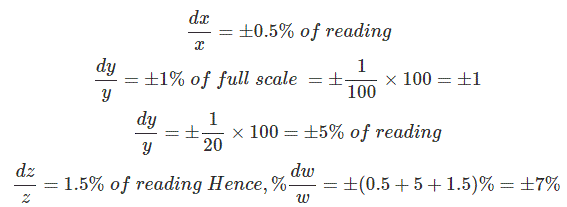# MCQs on Electrical And Electronics Measurements

##### Page 19 of 19. Go to page 1 2 3 4 5 6 7 8 9 10 11 12 13 14 15 16 17 18 19
01․ Which one of the following meters has maximum loading effect on the circuit under measurement ?
1000 Ω/volt
10 MΩ/volt
100 Ω/volt
1 MΩ/volt

Maximum loading effect takes place due to low sensitivity of the instrument.

02․ The inductance of a certain moving iron ammeter is expressed as L = 2+5θ-θ2 µH. The control spring torque is 2 × 10-6 Nm/radian. Find the deflection in radians for a current of 2A.
1
2
4
5

The deflecting torque of a moving iron instrument (T) = 0.5I2dL/dθ. I = 2A; T = 2 × 10-6 Nm/radian; dL/dθ = 5-2θ µH; So, 5-2θ = 1; θ = 2.

03․ A variable w is related to three other variables x, y and z as w = xy/z. The variables are measured with meters of accuracy ± 0.5% reading, ± 1% of full scale value and ± 1.5% reading. The actual readings of the three meters are 80, 20 and 50 with 100 being the full scale value for all three. The maximum uncertainty in the measurement of w will be
± 5%
± 6%
± 7%
± 8%04․ Assertion (A) : It is always desirable to take the reading of an indicating instrument very close to the full scale reading. Reason (R) : Accuracy of an indicating instrument is maximum at the full scale deflection and error increases as reading comes closer to the beginning of the scale.
A is true but R is false
A is false bur R is true
Both A and R are true and R is the correct explanation of A
Both A and R are true but R is NOT the correct explanation of A

Accuracy of an indicating instrument is maximum at the full scale deflection and error increases as reading comes closer to the beginning of the scale.

<<<171819